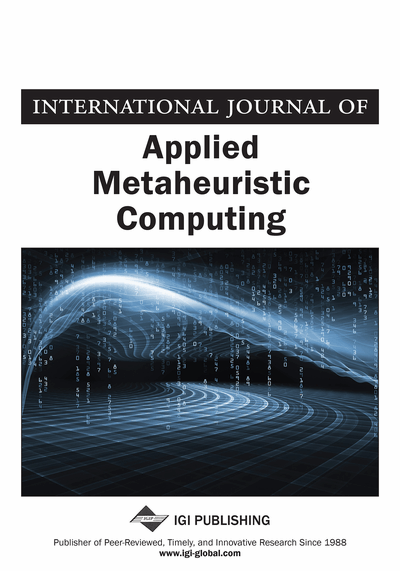# SODAC: A Simulation-Based Tool for the Optimal Design of Analog Circuits

Amin Sallem (LETI-ENIS, University of Sfax, Sfax, Tunisia), Mourad Fakhfakh (LETI-ENIS, University of Sfax, Sfax, Tunisia), Esteban Tlelo-Cuautle (Department of Electronics, Instituto Nacional de Astrofísica, Óptica y Electrónica, Tonantzintla, Puebla, Mexico) and Mourad Loulou (LETI-ENIS, University of Sfax, Sfax, Tunisia)
DOI: 10.4018/jamc.2012100104
Available
\$37.50
No Current Special Offers

## Abstract

It is of common use that analog designers start by optimizing the basic building bloc forming an active circuit in order to ‘optimally’ size the latter. Even though it is known a priori that the overall circuit performances will differ from the expected ones, due to the fact that the performances of the basic cells will (considerably) change because of the surrounding circuitry, such approach is very widely used. This is mainly due to the complexity of these ‘complex’ circuits. It has recently been shown that the simulation based sizing technique is a very interesting spare solution, since it allows avoiding the (very) ‘complex’ modeling task. In this paper the authors propose a simulation based optimizing tool that can handle both mono-objective and multi-objective optimization sizing problems. Viability and benefits of this tool are highlighted through some examples. Obtained results are compared to the ideal expected ones and to the ones that are obtained using the conventional approaches.
Article Preview
Top

## 1. Introduction

Analog circuit design and sizing is a very complicated and iterative process. Unlike its digital counterpart, it has not yet reached a mature stage. This is due to many reasons, mainly because the analog design field presents an extra large spectrum of circuit performances, constraints and tradeoffs. Designers generally count on their experience and on the use of iterative simulations to optimize their circuits. Therefore, there is a pressing need for analog circuit design automation (Gielen & Rutenbar, 2000). Recent advances in design automation have led to a gradual transition from the hand-calculation based design, namely knowledge-based design, to the optimization-based design. Knowledge-based approaches are characterized by the use of a predefined design plan describing how the circuit components must be sized to reach the optimum solution of the design problem (Shravan et al., 2011; Degrauwe et al., 1987; Harjani et al., 1989; El-Turky & Perry, 1989). On the other hand, the optimization-based approaches use an iterative search algorithm for solving the circuit’s sizing problem.

Actually, two different techniques can be adopted for solving such problem:

• The equation-based optimization technique, which uses symbolic (or semi-symbolic) expressions of both performances and constraints to evaluate the circuit’s performance(s) and to generate the optimal values of the circuit’s parameters. These equations can be derived manually (for small size problems), or by using a symbolic analyzer (for medium and large size problems), such as, OPASYN (Koh et al., 1990), STAIC (Harvey et al., 1992), CASCADES (Fakhfakh & Loulou, 2010). The advantage of this approach, i.e., the equation-based technique, mainly consists of the reduced computation time, since the evaluation of the performance is performed by direct evaluation of symbolic equations. However, generally such equations are marred by errors. These errors are due to the unavoidable use of simplified models of non-linear components, mainly transistors’ models.

• The simulation-based technique evaluates the circuit’s performances, as well as its constraints, using a circuit simulator, such as SPICE. Thus, one directly takes benefits of the simulator models without any need of simplifications. In other words, the final result corresponds exactly to the expected one (Guerra-Gomez et al., 2008, 2010)

In this paper we present in details ‘‘SODAC’’; a simulation-based optimization tool, and we highlight via some examples the advantages and the necessity of the use of such sizing/optimization tool. Comparisons with other approaches are given, and simulation results are presented to further highlight these statements.

The rest of the paper is structured as follows. In Section 2, we put the light on the proposed tool and highlight its capabilities. In Section 3, we present some application examples where we show the usefulness of such tool: First, we deal with optimizing performances of analog circuits, namely current conveyors. Second, we focus on the use of current conveyors as building blocs for the design of active circuits. In Section 4, we present details of the multi-objective module of SODAC and we show case its viability via two examples. Finally, concluding remarks are given in Section 5.

Top

## 2. The Simulation-Based Sizing/Optimization Technique

One of the key components in the simulation based optimization technique is the optimization bloc. The purpose of this optimizer is to find a set of parameters that optimizes the (set of) performance function(s) while satisfying a set of constraints (see expression (1)).MinimizeSubject to:and:(1) wherek, m, n and l denote numbers of objectives, inequality constraints, equality constraints and parameters, respectively.

## Complete Article List

Search this Journal:
Reset
Volume 13: 4 Issues (2022): 2 Released, 2 Forthcoming
Volume 12: 4 Issues (2021)
Volume 11: 4 Issues (2020)
Volume 10: 4 Issues (2019)
Volume 9: 4 Issues (2018)
Volume 8: 4 Issues (2017)
Volume 7: 4 Issues (2016)
Volume 6: 4 Issues (2015)
Volume 5: 4 Issues (2014)
Volume 4: 4 Issues (2013)
Volume 3: 4 Issues (2012)
Volume 2: 4 Issues (2011)
Volume 1: 4 Issues (2010)
View Complete Journal Contents Listing